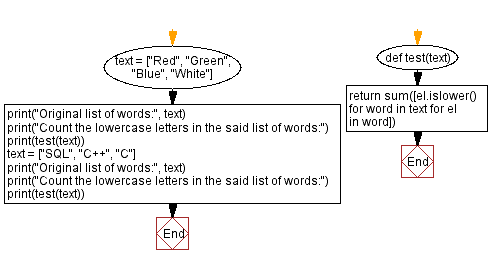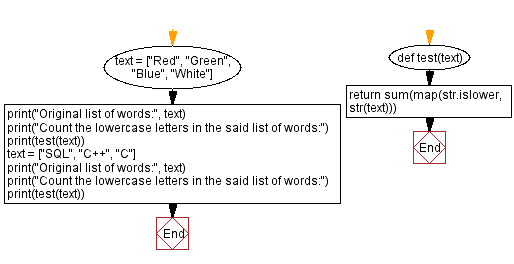﻿ Python: Count lowercase letters in a list of words

# Python Exercises: Count lowercase letters in a list of words

## Python List: Exercise - 274 with Solution

Write a Python program to count the lowercase letters in a given list of words.

In the Code tab is a function which is meant to return how many uppercase letters there are in a list of various words. Fix the list comprehension so that the code functions normally!.

Sample Data:
(["Red", "Green", "Blue", "White"]) -> 13
(["SQL", "C++", "C"]) -> 0

Sample Solution-1:

Python Code:

``````# Define a function named 'test' that takes a list of words 'text' as input.
def test(text):
# Use a list comprehension to count the lowercase letters in each word.
# Iterate through the words in 'text' (outer loop).
# Iterate through the characters in each word (inner loop).
# Count the lowercase characters by checking if each character is lowercase.
# Sum the count of lowercase characters for all words.
return sum([el.islower() for word in text for el in word])

# Define a list of words 'text'.
text = ["Red", "Green", "Blue", "White"]
print("Original list of words:", text)
# Call the 'test' function to count the lowercase letters in the list of words.
print("Count the lowercase letters in the said list of words:")
print(test(text))

# Define another list of words 'text'.
text = ["SQL", "C++", "C"]
print("\nOriginal list of words:", text)
# Call the 'test' function for the second list of words.
print("Count the lowercase letters in the said list of words:")
print(test(text))
```
```

Sample Output:

```Original list of words: ['Red', 'Green', 'Blue', 'White']
Count the lowercase letters in the said list of words:
13
Original list of words: ['SQL', 'C++', 'C']
Count the lowercase letters in the said list of words:
0
```

Flowchart:Sample Solution-2:

Python Code:

``````# Define a function named 'test' that takes a list 'text' as input.
def test(text):
# Use the 'map' function to apply the 'str.islower' method to each element in the input.
# The 'str.islower' method checks if a string contains only lowercase letters.
# The result is a list of boolean values indicating whether each element contains lowercase letters.
# Use 'sum' to count the 'True' values in the list (count of lowercase elements).
return sum(map(str.islower, str(text)))

# Define a list of words 'text'.
text = ["Red", "Green", "Blue", "White"]
print("Original list of words:", text)
# Call the 'test' function to count the lowercase letters in the list of words.
print("Count the lowercase letters in the said list of words:")
print(test(text))

# Define another list of words 'text'.
text = ["SQL", "C++", "C"]
print("\nOriginal list of words:", text)
# Call the 'test' function for the second list of words.
print("Count the lowercase letters in the said list of words:")
print(test(text))
```
```

Sample Output:

```Original list of words: ['Red', 'Green', 'Blue', 'White']
Count the lowercase letters in the said list of words:
13
Original list of words: ['SQL', 'C++', 'C']
Count the lowercase letters in the said list of words:
0
```

Flowchart:Python Code Editor:

Previous Python Exercise: Divide a list of integers with the same sum value.
Next Python Exercise: Sum of all list elements except current element.

What is the difficulty level of this exercise?

Test your Programming skills with w3resource's quiz.

﻿